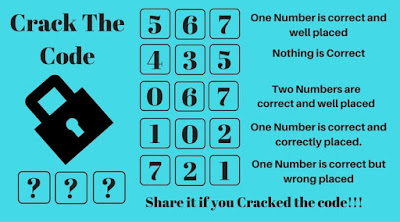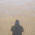## Friday, August 30, 2019

Here are some Critical Thinking Puzzles in which one has to think logical to crack the 3 digits which will open the door. Earlier we have published Logical Crack the Code Puzzles and Mathematical Crack the Code Puzzles. Today's Crack the Code Puzzles are logical i.e. instead of any mathematical calculations, it will require critical logical thinking to solve these puzzles.
Crack the Code are the puzzles in which there is lock which has 3 digit key to open it. There are 3 digit hints given and it is told how many of these three digits are correct. If these numbers are correct then how many of these correct numbers are correctly placed.
Answers of these Crack the Code Puzzles are given at the end. Do try to crack this code by yourself and use the given answers only to check your solutions.3. Can you Crack the Code?4. Open the Lock by Cracking the Logical Code.

Check out some more Logical Brain Teasers as mentioned below to keep challenging your mind
List of Logical Brain Teasers

1. Out of Box Thinking Fun Brain Teasers:  It contains the mixed Logical and Mathematical Puzzles which will require out of box thinking of solving these puzzles.

2. Interesting Brain Teasers to Challenge your Mind: It contains different types of brains teasers e.g. Mathematical Puzzles, Logical Brain Teaser and Picture Puzzles.

3. Fun Brain Teasers: You can solve many Brain Cracking Fun Maths Logic Problem which will help you to increase your logical and mathematical brainpower.

4. Quick Fun Brain Teasers with answers: Do checkout Fun Brain Teasers which you have to answer very quickly. Let us see how many of these brain teasers you can solve correctly?

1.1.